# keras与tensorboard结合使用

keras的在训练（fit）的过程中，显式地生成log日志；使用tf的tensorboard来解析这个log日志，并且通过网站的形式显示出来。

fit的时候加上callbacks=[TensorBoard(log_dir='./tmp/log')] 将运行的结果保存在'./tmp/log'下。执行tensorboard 命令的时候指明： --logdir=./temp/log

epoch是很行的选择。它使用的是

# Fit the model on the batches generated by datagen.flow().
model.fit_generator(datagen.flow(x_train, y_train,
batch_size=batch_size),
epochs=epochs,
validation_data=(x_test, y_test),
workers=4)

model.fit_generator(datagen.flow(x_train, y_train,
batch_size=batch_size),
epochs=epochs,
validation_data=(x_test, y_test),
workers=4,
callbacks=[TensorBoard(log_dir='./tmp/log')])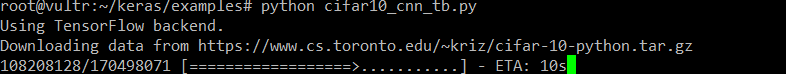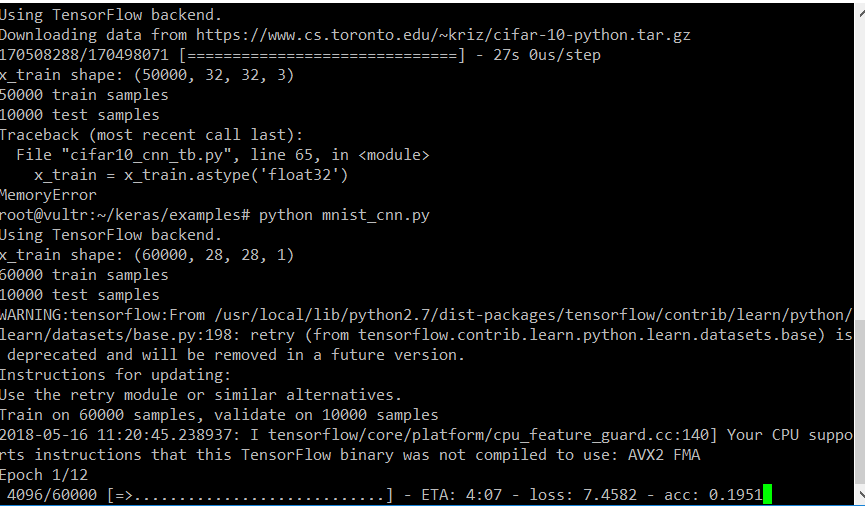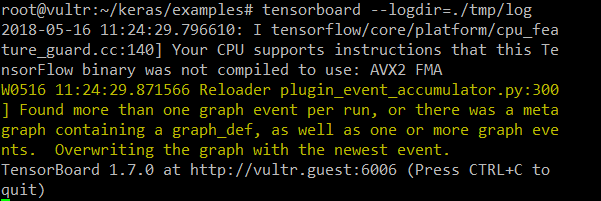~/keras/examples 目录下进行的，有绝对地址的说法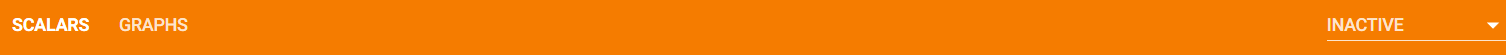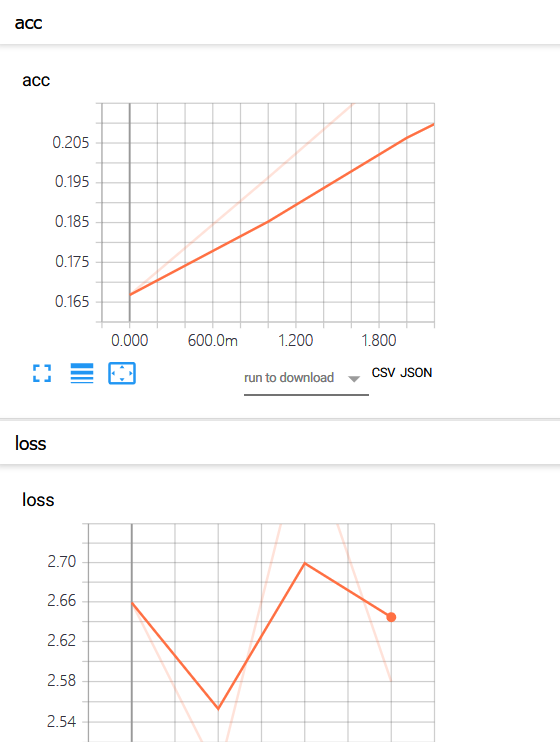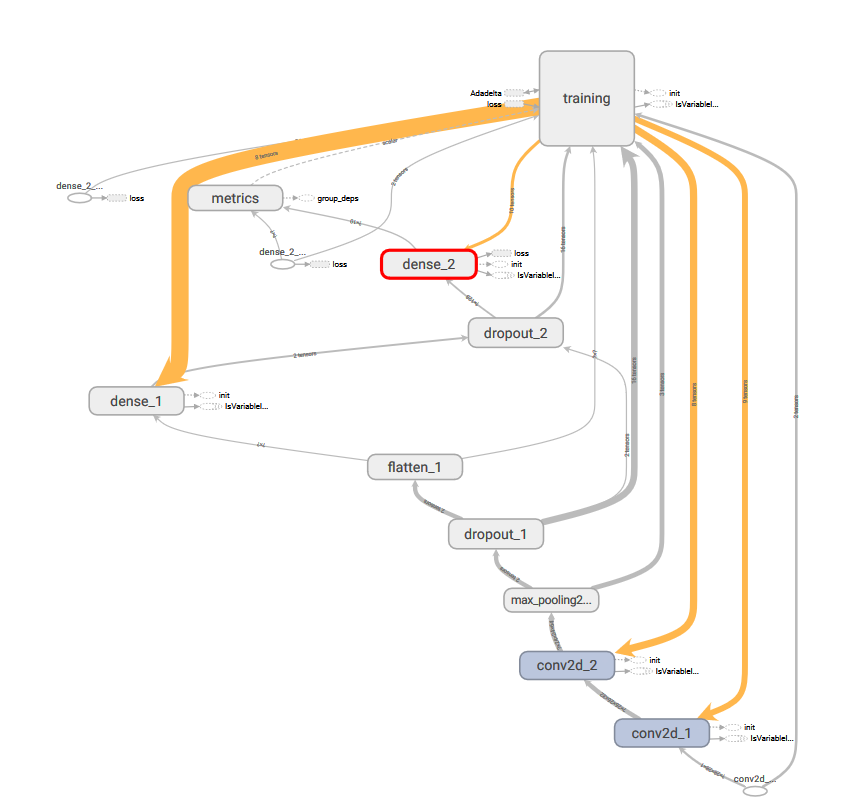posted @ 2018-05-16 19:57 jsxyhelu 阅读(...) 评论(...) 编辑 收藏